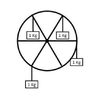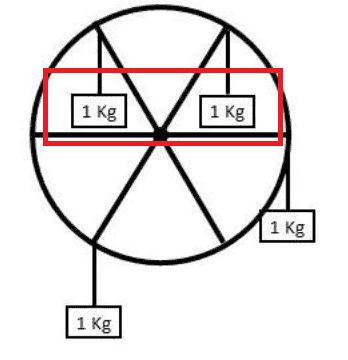# 6 spoke wheel torque

paulimerci
Homework Statement:
A 6 spoke wheel with a radius of 2 meters has 4 - 1 Kg masses hanging from the point where the spoke meets the rim as shown. What would be the net torque acting on the wheel?
Relevant Equations:
Applying Rotational equlibrium, F1d1 + F2d2 = F3d3 + F4d4
F_{1}d_{1} + F_{2}d_{2} = F_{3}d_{3} + F_{4}d_{4}
m_{1} gR cos 60 + m_{2}gR cos 60 = m_{3}gR cos 60 + m_{4}gR sin 90
m1 = m2= m3= m4= m
R1=R2=R3=R4=R
\sigma\tau = sin 90 - cos 60 = 0.5 Nm.
Have I done this right?

#### Attachments

•Screen Shot 2022-10-28 at 10.59.26 PM.png
12.9 KB · Views: 15

Homework Helper
Gold Member
2022 Award
Homework Statement:: A 6 spoke wheel with a radius of 2 meters has 4 - 1 Kg masses hanging from the point where the spoke meets the rim as shown. What would be the net torque acting on the wheel?
Relevant Equations:: Applying Rotational equlibrium, F1d1 + F2d2 = F3d3 + F4d4

R1=R2=R3=R4=R
You only have R as variable in your torque equation
m_{1} gR cos 60 + m_{2}gR cos 60 = m_{3}gR cos 60 + m_{4}gR sin 90

You should be able to argue that the torques generated by these two masses cancel straight away•topsquark
Staff Emeritus
Homework Helper
Gold Member
The result is correct. The reasoning is not. You cannot apply rotational equilibrium because there is a net torque (it is what you are asked to find!)
Your result also does not follow from the zero net torque equation.

•topsquark and paulimerci
paulimerci
yes, I did that. Thanks!

paulimerci
The result is correct. The reasoning is not. You cannot apply rotational equilibrium because there is a net torque (it is what you are asked to find!)
Your result also does not follow from the zero net torque equation.
Thanks!

paulimerci
Thanks!

\sigma \tau = m_{1} gR cos 60 + m_{2}gR cos 60 - m_{3}gR cos 60 - m_{4}gR sin 90 = -sin 90 + cos 60 = - 0.5 Nm. Have I done it right now? It looks like I got negative torque.

Homework Helper
Gold Member
2022 Award
##\Sigma \tau = m_{1} gR cos 60 + m_{2}gR cos 60 - m_{3}gR cos 60 - m_{4}gR sin 90 = -sin 90 + cos 60 = - 0.5 ##Nm. Have I done it right now? It looks like I got negative torque.
It's negative because it is clockwise.
But your answer is wrong numerically. What is the weight of a 1kg mass at Earth's surface?

Note it is \Sigma for ##\Sigma##, and on this platform the LaTeX has to be enclosed in double hashes (# #, without the space), or double \$ if you want it on a separate line.

•topsquark
paulimerci
It’s 9.81kgm/s2

Gold Member
Homework Statement:: A 6 spoke wheel with a radius of 2 meters has 4 - 1 Kg masses hanging from the point where the spoke meets the rim as shown. What would be the net torque acting on the wheel?
Relevant Equations:: Applying Rotational equlibrium, F1d1 + F2d2 = F3d3 + F4d4

m1 = m2= m3= m4= m
R1=R2=R3=R4=R
\sigma\tau = sin 90 - cos 60 = 0.5 Nm.
Have I done this right?
Are you cancelling out the m's and R's.
What should be the final equation to determine the torque?

your torque can be negative, or positive.
You did not up front specify which direction - clockwise or counterclockwise - is in the positive direction.

Although, implicitly, the positive direction can be gleamed from your balance of torques equation as being counterclockwise.

paulimerci
It’s 9.81kgm/s2
Oh. I forgot to include g

paulimerci
I got the answer as -9.8Nm.

•